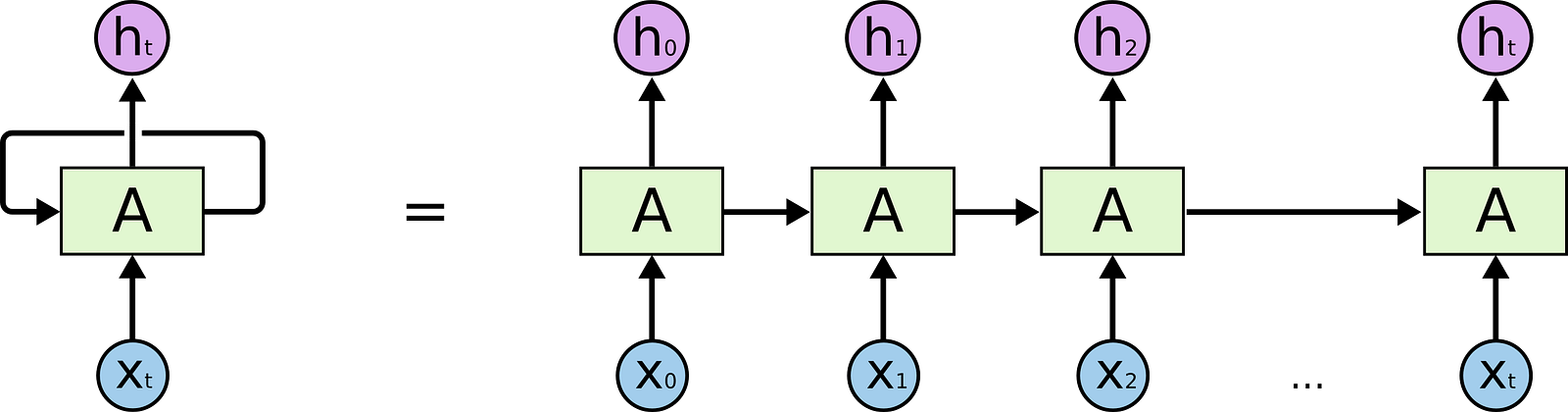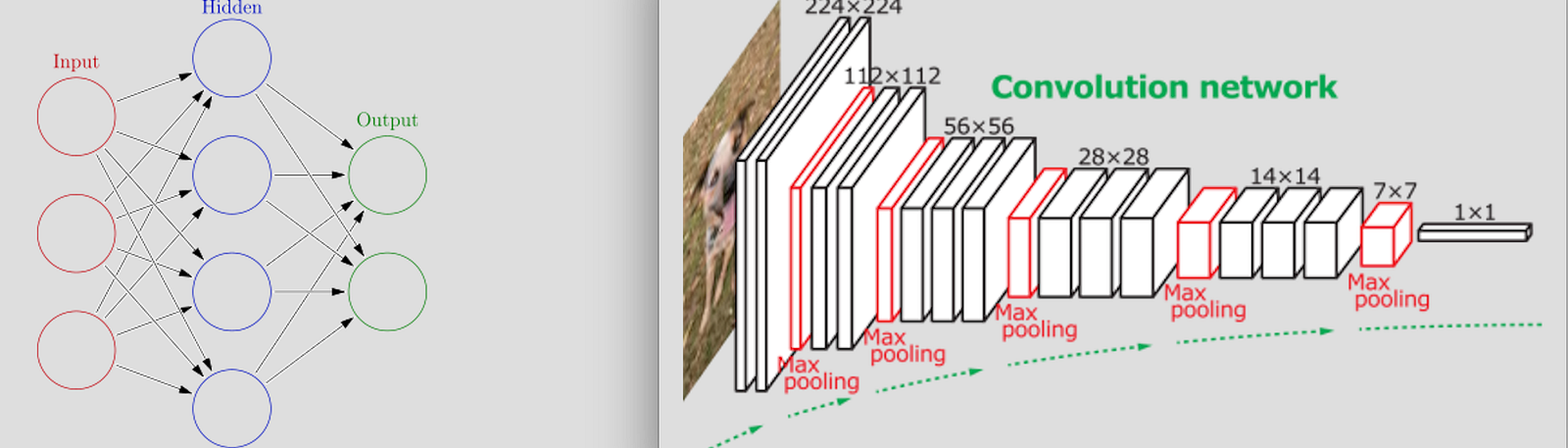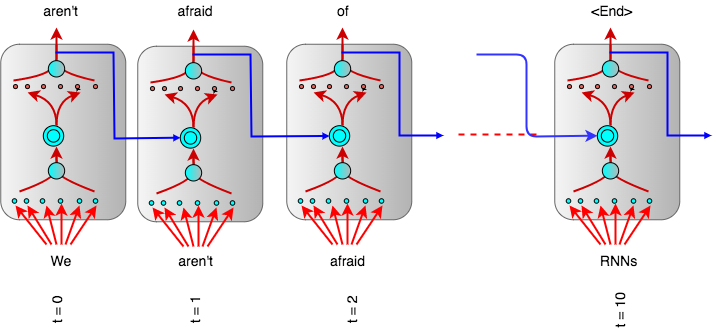# Unmasking a vanilla RNN: What lies beneath?

RNNs have a reputation for being rather hard to understand. They are truly a bit mysterious, and can often seem inscrutable to the new learner. When one starts talking about LSTMs, or the rolled/unrolled versions of the RNN architectures, these discussions can definitely send some scurrying faster than the ghost that lived in Michelle Peifer’s home.

I think the reason for this is that there exists few materials that expose the internals of a RNN in a way that is easy to visualize. Without having a good mental image of what the building block is, I find myself times and often struggling to understand the same thing over and over again.

### Core APIs

Now at the core of any RNN architecture is a simple RNN cell or its variant. This is what it takes to create a RNN Cell in PyTorch:

`rnn_pytorch = nn.RNN(input_size=10, hidden_size=20)`

and this is what it takes to create an RNN Cell in Tensorflow:

`rnn_tensorflow = tf.contrib.BasicRNNCell(num_units=20)`

Notice the usage of the word Cell. Somehow, the term cell seems very alien in neural networks, where we’re used to visualizing individual neuron and/or their arrangements in space with mutual connections. How does a RNN cell relate to the floating collections of individual neurons that we’re much used to in neural nets? This is the visual we’ll explore in the rest of this blog.

A RNN cell should simply be thought of as a unit of the overall network that encapsulates certain parts of it in one homogeneous block. In most blogs and papers, an RNN cell (block) is shown as the follows:Typically how a simple RNN is shown (Left: Canonical form, Right: Unrolled form)

which is very different from how traditional fully-connected (FC) and Convolution networks (conv-nets) are depicted.Typical view of Neural Networks (Left: Fully-Connected, Right: Convolution Networks)

### Under the hood:

The best way to intimately understand the structure of a RNN cell maybe by creating one ourself! Here’s the basic RNN cell created from a scratch in PyTorch.

`class CharLoopModel(nn.Module):    # This is an RNN!`
`    def __init__(self, vocab_size, in, h):        super().__init__()`
`        self.h = h        self.e = nn.Embedding(vocab_size, in)        self.l_in = nn.Linear(in, h)        self.l_hidden = nn.Linear(h, h)        self.l_out = nn.Linear(h, vocab_size)            def forward(self, *cs):`
`        '''        cs (list(list(int))): input signals to propagate forward.        Example: ((23,32,34), (12,24,23), (12, 45,23))        '''                bs = cs.size(0)        hidden_state = Variable(torch.zeros(bs, self.h)).cuda()        for c in cs:            inp = F.relu(self.l_in(self.e(c)))            hidden_state = F.tanh(self.l_hidden(hidden_state+inp))                return F.log_softmax(self.l_out(hidden_state), dim=-1)`

The code above gives us a very good idea of the internals of the RNN Cell. Note that the two chief constituents that participate to form a RNN are the self.l_in and self.l_hidden layers. Via the analysis of the __init__ and the forward method, we can equivalently represent the composition as the following diagram:

From the figure above, it should be evident that an RNN Cell is really a set of two fully-connected linear layers. Both these layers have ‘h’ neurons, where ‘h’ is the user-specified dimension of the hidden state. The only special thing that happens internally is the addition of the “hidden state” to the output from the first layer. That’s it!!

The hidden-state forms a recurrent connection within the layer (and from where the RNN cell derives it names). In typical usage, the recurrent connection allows the network to remember what it learned in the previous step. RNNs have been used in many time or sequence dependent problems for precisely this reason.

### Unrolled RNN Graph:

The unrolled RNN graph of the same can be imagined with very little additional effort. It shows how the recurrent connection flows from one-step to another allowing the RNN Cell to exhibit its memory-like characteristic.

Training the RNN is usually executed one step at a time. Thus, RNNs are also notorious for being difficult to train because they cannot utilize the fully parallel architectures of modern hardware. By the nature of the problem (and proposed solution), the computation can only proceed one step at a time forward. In the same way, back-propagation through a RNN cell involves evaluating the gradient at the very last time-step, and propagating it backwards one time-step back at a time.An RNN Cell’s computational graph showed unrolled over time

### Finally,

RNNs have been used extensively for modeling problems that are time or sequence dependent. Their prowesses for problems, especially in the language-modeling domain such as machine translation, speech recognition, and text summarization, have become far superior to any traditional methods.

At its heart, an RNN cell (or any of its variant) is really a composition of linear dense layers that introduce recurrence via some moderated connections. Actually, modern RNN architectures rarely every use the basic RNN cell that we studied above. Rather, they most often use the LSTM cell, which is just a kind of RNN cell that introduce many more intra-recurrent connections. Ultimately, having a good understanding of the structure of the basic RNN cell can often help guide to more intuitive understanding of more complex cells and how they function.

### Acknowledgement

Once again, I owe Jeremy’s fastai course for many of the insights that were provided in this post, including the core code for the PyTorch implementation of the RNN from a scratch. The accompany source code on github goes on to demonstrate the usage of this code for a sample text corpus, as well as explore additional variations of the RNN.

I had also previously implemented the RNN via pure tensorflow, but the end-to-end implementation, including the demonstration, is much more involved herein. The key to this implementation was using tensorflow’s scan method, which essentially performed the dynamic unrolling of the computational graph, thus obfuscating the matter quite a bit!